Ask your WordPress questions! Pay money and get answers fast!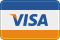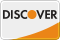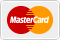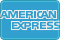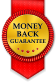# Calculating probability over several iterations? WordPress

• SOLVED

I am facing a tight deadline.

This is a PHP and math question, not a WordPress question. But I ask it here as I know you are all good with PHP, and some of you are good at math.

I need the PHP code to resolve questions like:

If event X is 20% likely on one attempt, then what is the cumulative chance of event X happening after 60 attempts?

If event Y is 90% likely on the first iteration, then how likely is it to have happened at all after 200 iterations?

For instance, death. If you are 15 years old, and you live in the USA, then your chance of dying during the next 12 months is 1/2000. If people never aged, but always had the health of a 15 year old, than the average age of death would be 1,200 years. That is, if your death has a 1/2000 chance of happening during the next year, then after 1,200 years you face just over a 50% chance of death.

Anyone have an easy PHP function for this?

2011-08-10

I think this is the answer:

```function my_probability(\$a, \$b) { return (1.0 - pow(1.0 - \$a, \$b)); }```

For your input 1/2000, and 1200, it gives a probability of 45%.

2011-08-10

The correct answer is the following:

<strong>Math:</strong>

Event X: probability of occurring: 0.2, probability of not occurring: 0.8

Probability that even X occurs on try 1: 0.2
Probability that even X occurs on try 2: 0.8 * 0.2 = 0.16 (ie: not occurring on try 1 AND occurring on try 2)
Probability that even X occurs on try 3: 0.8 * 0.8 * 0.2 = 0.128 (ie: not occurring on try 1 AND not occurring on try 2 AND occurring on try 3)

So, cumulative probability that it happens with 3 tries: 0.2 + 0.16 + 0.128 = 0.488 (happens on try one OR happens on try 2 OR happens or try 3) which is 48,8%

The iteration is, where P is the probability of the event and N the number of iterations:
P + P * (1 - P ) + P * (1 - P)² + .. + P * (1 - P) ^ (N - 1)